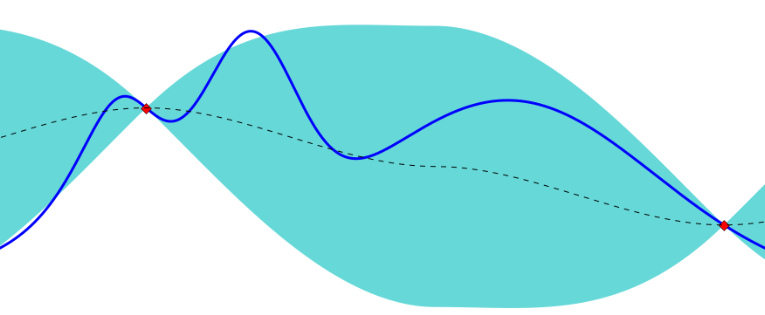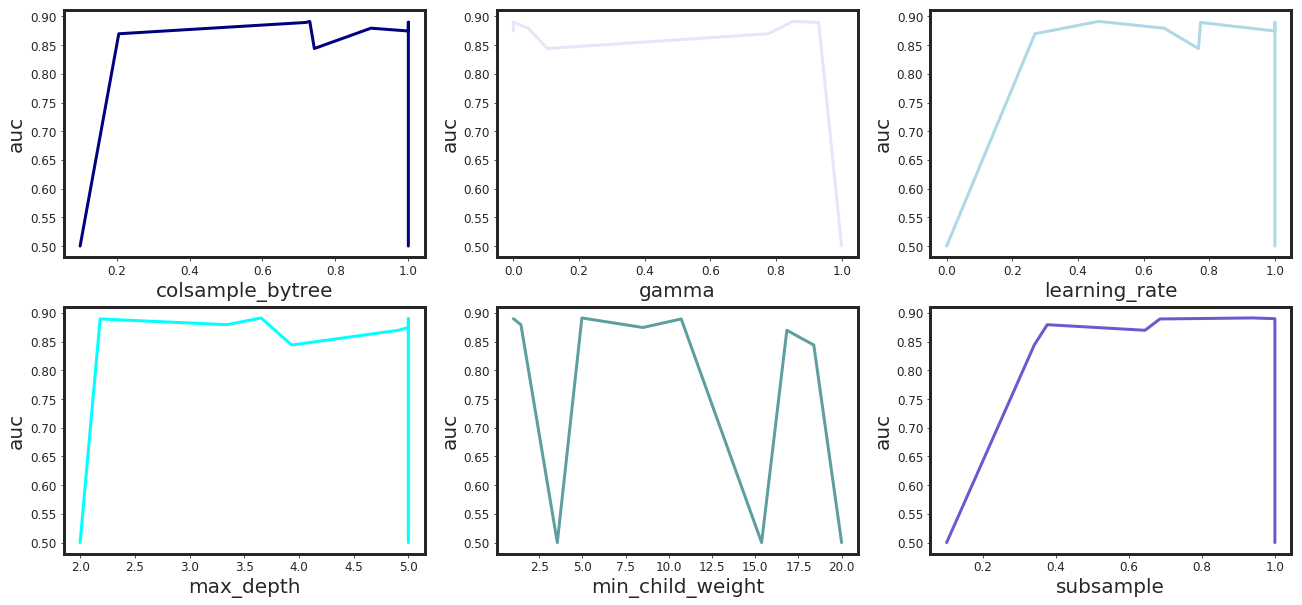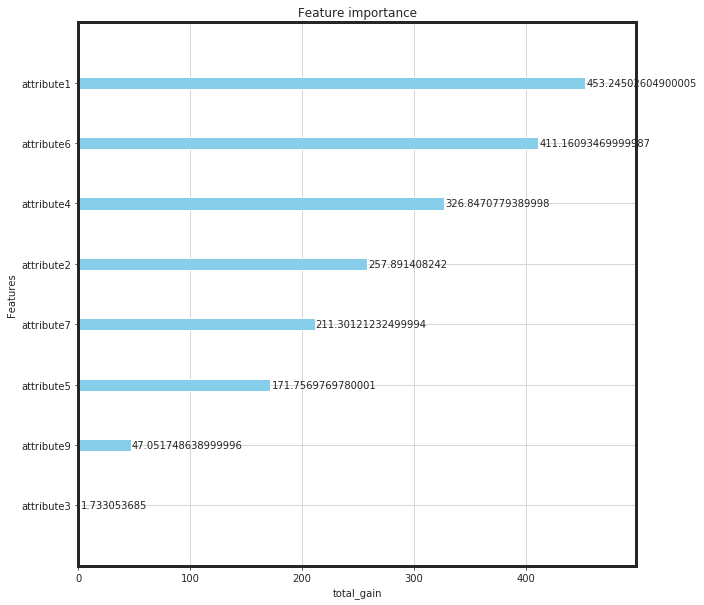## Gaussian processes (GPs) provide a principled, practical, and probabilistic approach in machine learning. GPs simply have an essential assumption that similar inputs give similar outputs. This simple and weak prior are actually very sensible for the effects of hyper-parameters. GPs are able to learn for each input dimension what the appropriate scale is for measuring similarity. GPs predict a Gaussian distribution of values rather than just predicting a single value. Bayesian optimization is a constrained global optimization approach built upon Bayesian inference and Gaussian process models to find the maximum value of an unknown function in the most efficient ways (less iterations).

``````# Loading libraries
from bayes_opt import BayesianOptimization as bo
import xgboost as xgb
import numpy as np
import pandas as pd
import matplotlib.pyplot as plt
from sklearn.preprocessing import scale
import warnings
warnings.simplefilter("ignore")
from IPython.core.display import display, HTML
display(HTML("<style>.container { width:95% !important; }</style>"))
import seaborn as sns
sns.set_style("ticks")
%matplotlib inline
``````

# Bayesian Optimization

``````def _my_bayesian_optimization(X, Y, n_iter = 5,
init_points = 5,
acq = "ei",
num_boost_round = 1000,
nfold = 10,
stratified = True,
metrics = ("auc"),
early_stopping_rounds = 20,
seed = 1367,
shuffle = True,
show_stdv = False,
pbounds = None,
importance_type = "total_gain",
callbacks = False,
verbose_eval = False):
"""
a function to run bayesian optimization for xgboost
input parameters:
X: features (pandas dataframe or numpy array)
Y: targets (1D array or list)
n_iter: total number of bayesian iterations (default = 5)
init_points: total initial points of optimization (default = 5)
acq
num_boost_rounds: max number of boosting rounds, (default = 1000)
stratified: stratificaiton of the targets (default = True)
metrics: classification/regression metrics (default = ("auc))
early_stopping_rounds: the criteria for stopping if the test metric is not improved (default = 20)
seed: random seed (default = 1367)
shuffle: shuffling the data (default = True)
show_stdv = showing standard deviation of cv results (default = False)
pbounds = set of parameters for bayesian optimization of xgboost cv
(default_params = {
"eval_metric" : "auc",
"tree_method": "hist",
"objective" : "binary:logistic",
"learning_rate" : 0.05,
"max_depth": 2,
"min_child_weight": 1,
"gamma" : 0.0,
"reg_alpha" : 0.0,
"reg_lambda" : 1.0,
"subsample" : 0.9,
"max_delta_step": 1,
"silent" : 1,
"scale_pos_weight" : 1
}
)
importance_type = importance type of xgboost as string (default = "total_gain")
the other options will be "weight", "gain", "cover", and "total_cover"
callbacks = printing callbacks for xgboost cv
(defaults = False, if True: [xgb.callback.print_evaluation(show_stdv = show_stdv),
xgb.callback.early_stop(early_stopping_rounds)])
verbose_eval : a flag to show the result during train on train/test sets (default = False)
outputs:
df_res: the parameters related to the best performance
xgb_params: a dictionary of the best parameters of xgboost

"""

# callback flag
if(callbacks == True):
callbacks = [xgb.callback.print_evaluation(show_stdv = show_stdv),
xgb.callback.early_stop(early_stopping_rounds)]
else:
callbacks = None

# pbounds
default_pbounds = {"max_depth" : (2, 5),
"learning_rate" : (0, 1),
"min_child_weight" : (1, 20),
"subsample" : (0.1, 1),
"gamma": (0, 1),
"colsample_bytree": (0.1, 1.0)
}

# updating the default parameters of the pbounds
if pbounds is not None:
for key, val in pbounds.items():
default_pbounds[key] = val

def __xgb_eval(learning_rate,
max_depth,
gamma,
colsample_bytree,
min_child_weight,
subsample):

params = {"eval_metric" : "auc",
"tree_method": "hist",
"objective" : "binary:logistic",
"max_delta_step": 1,
"silent" : 1,
"scale_pos_weight" : 1,
"reg_alpha" : 0.0,
"reg_lambda" : 1.0,
"learning_rate" : learning_rate,
"max_depth": int(max_depth),
"min_child_weight": min_child_weight,
"gamma" : gamma,
"subsample" : subsample,
"colsample_bytree" : colsample_bytree
}
dtrain = xgb.DMatrix(data = X, label = Y)
cv_result = xgb.cv(params = params,
dtrain = dtrain,
num_boost_round = num_boost_round,
nfold = nfold,
stratified = stratified,
metrics = metrics,
early_stopping_rounds = early_stopping_rounds,
seed = seed,
verbose_eval = verbose_eval,
shuffle = shuffle,
callbacks = callbacks)

return cv_result.iloc[-1]

xgb_bo = bo(__xgb_eval, default_pbounds, random_state = seed, verbose = 2)
xgb_bo.maximize(init_points = init_points, n_iter = n_iter, acq = acq)

targets = []
for i, rs in enumerate(xgb_bo.res):
targets.append(rs["target"])
best_params = xgb_bo.res[targets.index(max(targets))]["params"]
best_params["max_depth"] = int(best_params["max_depth"])

xgb_params = {"eval_metric" : "auc",
"tree_method": "hist",
"objective" : "binary:logistic",
"max_delta_step": 1,
"silent" : 1,
"scale_pos_weight" : 1,
"reg_alpha" : 0.0,
"reg_lambda" : 1.0,
"learning_rate" : 0.05,
"max_depth": 2,
"min_child_weight": 5,
"gamma" : 0.0,
"subsample" : 1.0,
"colsample_bytree" : 0.9
}
for key, val in best_params.items():
xgb_params[key] = val

dtrain = xgb.DMatrix(data = X, label = Y)
bst = xgb.train(params = xgb_params,
dtrain = dtrain,
num_boost_round = num_boost_round)

# build results dataframe
frames = []
for idx, res in enumerate(xgb_bo.res):
d = res['params']
d[metrics] = res["target"]
frames.append(pd.DataFrame(data = d, index = [idx]))

res_df = pd.concat(frames)

print(F"-*-*-*-*-*-* Optimization Results -*-*-*-*-*-*")
display(res_df)

# Plotting
import matplotlib as mpl

mpl.rcParams['axes.linewidth'] = 3
mpl.rcParams['lines.linewidth'] = 3
cols = [col for col in res_df.columns.tolist() if col != "auc"]
ip = 1
plt.figure(figsize = (22, 10))
colors = ["navy", "lavender", "lightblue", "cyan", "cadetblue", "slateblue"]
for col in cols:
res_df.sort_values(by = col, inplace=True)
plt.subplot(2,3,ip)
plt.plot(res_df.loc[:, col], res_df.loc[:, metrics], color = colors[ip-1])
plt.xlabel(F"{col}", fontsize = 20)
plt.ylabel(F"{metrics}", fontsize = 20)
plt.tick_params(axis='both', which='major', labelsize = 12)
ip += 1
plt.show()

print(F"-*-*-*-*-*-* Best Performance -*-*-*-*-*-*")
display(res_df.loc[res_df[metrics] == res_df[metrics].max(), :])

from xgboost import plot_importance
from pylab import rcParams
rcParams['figure.figsize'] = (10,10)
plot_importance(bst, importance_type = importance_type, color = "skyblue", xlabel = importance_type)
plt.show()

return res_df, xgb_params

def _x_y_maker(df, target, to_drop):
"""
A function to receive the dataframe and make the X, and Y matrices:
----------
parameters

input: dataframe df
string target (class label)
list[string] to_drop (list of columns need to be dropped)

output: dataframe Feature matrix (df_X)
Target vector (Y)
"""
Targets = df[target]
to_drop.append(target)
Features = df.drop(to_drop, axis = 1)
Scaled_Features = scale(Features.values)
df_X = pd.DataFrame(data = Scaled_Features, columns = Features.columns.tolist())
Y = Targets.values

return df_X, Y

``````

``````dateparser = lambda x: pd.datetime.strptime(x, "%Y-%m-%d")
parse_dates = ["date"],
date_parser = dateparser,
encoding = "cp1252")

print("Shape: {}".format(df_raw.shape))
print("Prevalence = {:.3f}%".format(df_raw["failure"].sum()/df_raw.shape * 100))
``````
``````Shape: (124494, 12)
Prevalence = 0.085%
``````
``````df_raw.head()
``````
date device failure attribute1 attribute2 attribute3 attribute4 attribute5 attribute6 attribute7 attribute8 attribute9
0 2015-01-01 S1F01085 0 215630672 56 0 52 6 407438 0 0 7
1 2015-01-01 S1F0166B 0 61370680 0 3 0 6 403174 0 0 0
2 2015-01-01 S1F01E6Y 0 173295968 0 0 0 12 237394 0 0 0
3 2015-01-01 S1F01JE0 0 79694024 0 0 0 6 410186 0 0 0
4 2015-01-01 S1F01R2B 0 135970480 0 0 0 15 313173 0 0 3
``````df = df_raw.copy()
target = "failure"
to_drop = ["date", "device", "attribute8"]
df_X, Y = _x_y_maker(df, target, to_drop)
``````
``````df_res, params = _my_bayesian_optimization(df_X, Y)
``````
``````|   iter    |  target   | colsam... |   gamma   | learni... | max_depth | min_ch... | subsample |
-------------------------------------------------------------------------------------------------
| [0m 1       [0m | [0m 0.8795  [0m | [0m 0.8975  [0m | [0m 0.04571 [0m | [0m 0.6628  [0m | [0m 3.343   [0m | [0m 1.436   [0m | [0m 0.3758  [0m |
| [0m 2       [0m | [0m 0.844   [0m | [0m 0.7422  [0m | [0m 0.1034  [0m | [0m 0.7671  [0m | [0m 3.934   [0m | [0m 18.39   [0m | [0m 0.3402  [0m |
| [0m 3       [0m | [0m 0.8697  [0m | [0m 0.206   [0m | [0m 0.7772  [0m | [0m 0.269   [0m | [0m 4.909   [0m | [0m 16.83   [0m | [0m 0.6439  [0m |
| [95m 4       [0m | [95m 0.8894  [0m | [95m 0.7187  [0m | [95m 0.9302  [0m | [95m 0.7736  [0m | [95m 2.183   [0m | [95m 10.72   [0m | [95m 0.6848  [0m |
| [95m 5       [0m | [95m 0.8912  [0m | [95m 0.7297  [0m | [95m 0.8513  [0m | [95m 0.4627  [0m | [95m 3.654   [0m | [95m 4.965   [0m | [95m 0.9395  [0m |
| [0m 6       [0m | [0m 0.5     [0m | [0m 0.1     [0m | [0m 1.0     [0m | [0m 0.0     [0m | [0m 2.0     [0m | [0m 3.543   [0m | [0m 0.1     [0m |
| [0m 7       [0m | [0m 0.8746  [0m | [0m 1.0     [0m | [0m 0.0     [0m | [0m 1.0     [0m | [0m 5.0     [0m | [0m 8.494   [0m | [0m 1.0     [0m |
| [0m 8       [0m | [0m 0.8899  [0m | [0m 1.0     [0m | [0m 0.0     [0m | [0m 1.0     [0m | [0m 5.0     [0m | [0m 1.0     [0m | [0m 1.0     [0m |
| [0m 9       [0m | [0m 0.5     [0m | [0m 1.0     [0m | [0m 1.0     [0m | [0m 0.0     [0m | [0m 2.0     [0m | [0m 15.37   [0m | [0m 1.0     [0m |
| [0m 10      [0m | [0m 0.5     [0m | [0m 0.1     [0m | [0m 1.0     [0m | [0m 1.0     [0m | [0m 5.0     [0m | [0m 20.0    [0m | [0m 0.1     [0m |
=================================================================================================
-*-*-*-*-*-* Optimization Results -*-*-*-*-*-*
``````
colsample_bytree gamma learning_rate max_depth min_child_weight subsample auc
0 0.897530 0.045712 0.662807 3.343081 1.435660 0.375782 0.879458
1 0.742226 0.103395 0.767115 3.934126 18.388615 0.340209 0.844032
2 0.206024 0.777161 0.269010 4.908585 16.829513 0.643933 0.869726
3 0.718692 0.930205 0.773597 2.183002 10.716203 0.684811 0.889447
4 0.729727 0.851274 0.462704 3.654198 4.964748 0.939488 0.891218
5 0.100000 1.000000 0.000000 2.000000 3.542604 0.100000 0.500000
6 1.000000 0.000000 1.000000 5.000000 8.493812 1.000000 0.874567
7 1.000000 0.000000 1.000000 5.000000 1.000000 1.000000 0.889880
8 1.000000 1.000000 0.000000 2.000000 15.368715 1.000000 0.500000
9 0.100000 1.000000 1.000000 5.000000 20.000000 0.100000 0.500000``````-*-*-*-*-*-* Best Performance -*-*-*-*-*-*
``````
colsample_bytree gamma learning_rate max_depth min_child_weight subsample auc
4 0.729727 0.851274 0.462704 3.654198 4.964748 0.939488 0.891218# Best XGBoost Parameters

``````params
``````
``````{'eval_metric': 'auc',
'tree_method': 'hist',
'objective': 'binary:logistic',
'max_delta_step': 1,
'silent': 1,
``````